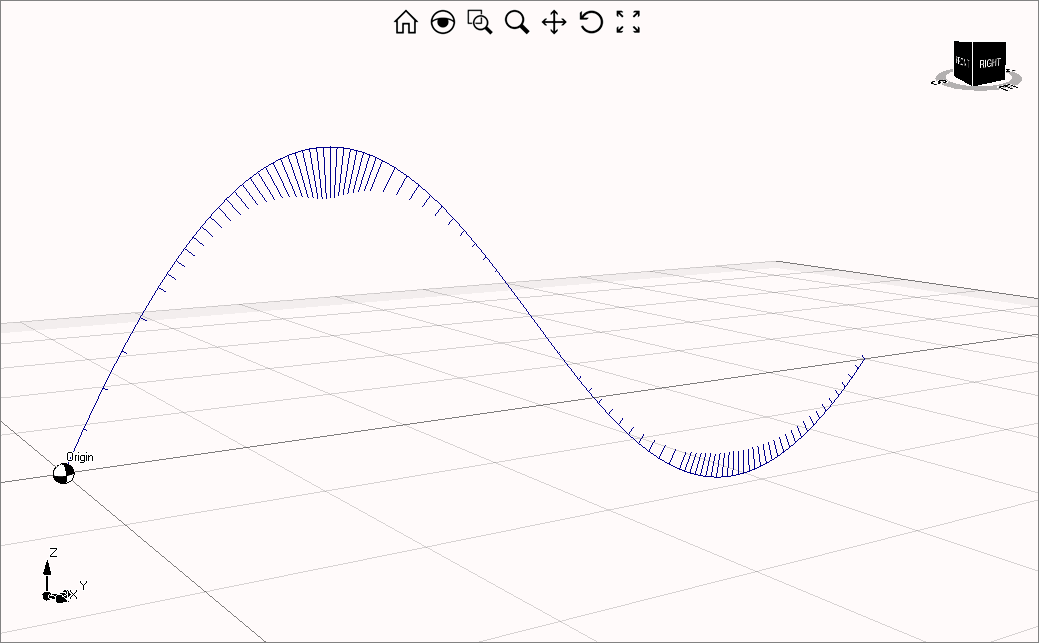# Curvature mapping for curves and surfaces

With the code samples reported in this article, you can display curvature mapping of surfaces and curves with Eyeshot.

The following picture shows the curvature mapping of a surface:Please note that there are different types of surface's curvature to display: Kappa1, Kappa2, Mean and Gauss. You can learn more about surface curvatures here.

The code used to display it is reported below:

``````
// Loft
List<Point3D> array = new List<Point3D>();

Curve first = Curve.GlobalInterpolation(array, 3);

array.Clear();

Curve second = Curve.GlobalInterpolation(array, 1);

array.Clear();

Curve third = Curve.GlobalInterpolation(array, 2);

array.Clear();

Curve fourth = Curve.GlobalInterpolation(array, 1);

array.Clear();

Curve fifth = Curve.GlobalInterpolation(array, 2);

Surface[] loft = Surface.Loft(new Curve[] { first, second, third, fourth, fifth }, 3);

model1.Legends.ColorTable = Legend.RedToBlue9;

foreach (Surface surf in loft)
{
surf.ComputeCurvatureMap(model1, model1.Legends, Surface.curvatureType.Kappa1);

surf.ShowCurvature = true;
}
model1.Legends.Visible = true;

``````

You can display also curve curvature mapping as shows the picture below:with the following code:

``````
List<Point3D> array = new List<Point3D>();

Curve curve = Curve.GlobalInterpolation(array, 3);

curve.ComputeCurvatureGraph(model1,20);

curve.ShowCurvature = true;

``````

Please note that function Curve.ComputeCurvatureGraph() needs a scale factor as input that is necessary to define the length of lines used to represent the curvature.